## conference papersJOURNAL OFAPPLIEDCRYSTALLOGRAPHY
ISSN: 1600-5767
Volume 40| Part s1| April 2007| Pages s250-s253

## The dynamic behavior of magnetic colloids in suspension

aHasylab/DESY, Notkestrasse 85, 22607 Hamburg, Germany, bESRF, BP 220, 38043 Grenoble Cedex, France, and cSaarland University, Physical Chemistry, 66123 Saarbrücken, Germany
*Correspondence e-mail: tina.autenrieth@desy.de

(Received 14 August 2006; accepted 23 February 2007; online 6 April 2007)

The dynamic behavior of magnetic colloidal particles in suspension is investigated. The particles of the core–shell colloid consist of a cobalt ferrite core embedded in a silica shell and are stabilized by surface charges. As the suspension is strongly opaque to visible light, it can not be probed by dynamic light scattering techniques as a result of strong multiple scattering as well as absorption effects. Therefore, the static and dynamic behavior is probed with small-angle X-ray scattering and X-ray photon correlation spectroscopy (XPCS), respectively. Using XPCS, we are able to study the diffusion coefficient of an opaque colloidal system as a function of the scattering vector. In this paper, we report on the behavior of the colloidal system in the absence of an external magnetic field, meaning that the magnetic moments of the particles are oriented randomly. We find no evidence for magnetic interactions in the static data, while the dynamic XPCS data deviate very significantly from the predictions of model calculations.

### 1. Introduction

The synthesis of highly defined colloidal particles with the ability to self-organize in suspension has provided us with interesting model systems for soft condensed matter. During the last few decades, mainly hard-sphere colloids (Pusey, 1991) or charge-stabilized particles have been investigated, with the common property of a purely repulsive and spherically symmetric interaction potential. In contrast, magnetic interactions are described by a dipole–dipole interaction potential,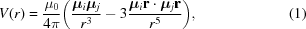where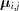denotes the magnetic moment of the particles, r the interparticle distance and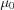the permeability. A suspension of magnetic colloidal particles, also known as a ferrofluid, consists of ferro- or ferrimagnetic single-domain particles with no long-range order between particles. Coating can prevent the particles from sticking to each other and the thermal (Brownian) motion keeps the particles suspended. If no external magnetic field is applied the magnetic moments are randomly oriented (Rosensweig, 1985).

Self-organization in colloidal matter is usually only observed if the particles display a high degree of monodispersity, which is increasingly difficult to achieve with decreasing particle diameter. This is the case for conventional commercially available ferrofluids consisting of magnetic particles with a typical diameter100 Å. For them the polydispersity is too high to observe any structured suspension. This problem can be overcome by synthesizing core–shell colloids. For an overall particle diameter of 400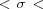1000 Å the polydispersity is low enough to permit self-organization to liquid-like or even crystalline ordered mesostructures. In contrast to hard-sphere colloids, where self-assembly occurs at relatively high volume fractions (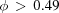), an ordering of charge-stabilized particles can be observed at volume fractions even below 0.01 (Härtl et al., 1999). The long-range interaction of charged particles is given by a screened Coulomb or Yukawa potential,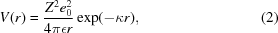with Z denoting the number of effective charges per particle,the permittivity andthe inverse Debye screening length, depending on the ionic strength in the suspension.

We prepared a water-based colloidal suspension combining electrostatic and magnetic dipole–dipole interactions. The synthesis results in a core–shell colloid bearing a magnetic cobalt ferrite core stabilized by a surface-charged silica shell. The magnetic moments of the core are randomly oriented as long as no magnetic field is applied. Thus we do not expect an influence of the magnetic properties on the static or the dynamic properties of the suspension. The direct interactions between the charged particles are purely repulsive and spherically symmetric. In addition, indirect interactions occur, mediated by shear waves resulting from the suspending medium, that can influence the dynamics of the system. The observed deviation of the dynamic data from theory (pairwise-additive approximation) points to a deficiency of the model for charge-stabilized suspensions at non-vanishing concentrations rather than to an effect of magnetic interactions. Results on the static behavior in the presence of a magnetic field are reported elsewhere (Wagner et al., 2006).

### 2. Theory, instrumentation and preparation

#### 2.1. Theory

Using small-angle X-ray scattering (SAXS), the time-averaged structure of complex liquids can be probed. The scattering intensity I(Q) of spherical particles is proportional to the product of the single-particle form factor P(Q) and the static structure factor S(Q),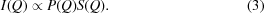The former describes the intraparticle structure, the latter the interaction between the particles. Here, Q is the modulus of the scattering vector, given by (4π/λ)sinθ, where λ is the wavelength of the incident X-rays and θ is half the scattering angle.

To probe the dynamics of an opaque system X-ray photon correlation spectroscopy (XPCS) is applied. This technique, using partially coherent X-rays, measures the intensity fluctuations I(Q,t) of the scattered light. In a homodyne experiment, the intensity autocorrelation function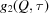is determined by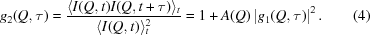The contrast A(Q) depends on the coherence properties of the source.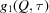is the field autocorrelation function [also called the intermediate scattering function f(Q,t)] that can be expressed for a diffusion process of non-interacting (Brownian) spherical particles by a single exponential decay,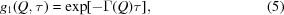with the relaxation constant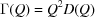. The diffusion coefficient D(Q) for non-interacting spherical particles with hydrodynamic radius RH suspended in a medium with the viscosityand temperature T reduces to the single-particle Stokes–Einstein self-diffusion coefficient D0: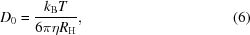kB is the Boltzmann constant. For interacting particles the diffusion process can be described by a time- and scattering-vector dependent diffusion coefficient D(Q,t). In the short-time limit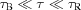, where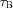describes the Brownian relaxation time and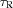the structural relaxation time (time for moving a distance comparable to the particle radius), one finds further that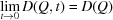and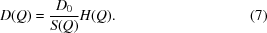H(Q) is the hydrodynamic function describing indirect hydrodynamic interactions mediated by the solvent. The hydrodynamic function H(Q) is connected to the diffusion tensors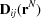via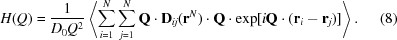This quantity contains the configuration-averaged effect of hydrodynamic interactions on the short-time dynamics. The pairwise-additive approximation is a two-particle approach for describing the hydrodynamics of highly charged and ultra dilute particle suspensions, proposed by Nägele & Baur (1997). For hard-sphere systems, the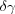expansion [a many-body approach proposed by Beenakker & Mazur (1984)] is an appropriate theory to describe hydrodynamics.

#### 2.2. Instrumentation

The SAXS and XPCS experiments were carried out at beamline ID10A of the European Synchrotron Radiation Facility (ESRF, France). The colloidal samples were placed in quartz capillaries (diameter = 1 mm) and illuminated with 8 keV X-rays (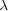= 1.54 Å) supplied by an Si(111) single-bounce monochromator (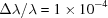). A platinum-coated mirror was set to a critical energy of 10 keV for harmonic rejection. A pinhole with 12 µm diameter was used to provide a partially coherent beam. We used fast avalanche photodiodes as detectors. For the XPCS experiment the detector was connected to a fast digital FLEX autocorrelator thus allowing the relaxation times at room temperature (T = 294 K) to be measured.

The dynamic behavior of a highly dilute suspension was investigated using dynamic light scattering (DLS) because the colloidal system is nearly translucent at such low particle concentrations. An ALV-5000E fast correlator (ALV Laservertriebsgesellschaft, Germany) was used to measure the correlation functions with a 35 mW He–Ne laser as a coherent light source (Coherent Inc., USA). The measurements were performed at T = 298 K and detected by a system consisting of a monomode cable with two cross-correlated photomultiplier channels.

Magnetization measurements were performed with a vibrating sample magnetometer (VSM, Oxford Instruments, UK).

#### 2.3. Synthesis of the core–shell colloids

The colloidal particles were prepared in a two-step synthesis that is described in detail elsewhere (Wagner et al., 2002). First, the magnetic core consisting of cobalt ferrite is precipitated from stoichiometric amounts of the corresponding cobalt and iron salts, according to Massart's method (Massart, 1981). The resulting magnetic nanoparticles are charge-stabilized in water by nitrate ions and have to be transferred in an ethanol/ammonia medium (Philipse et al., 1994) to continue with the second preparation step. Here, the silica shell is prepared via a Stöber polycondensation process starting from tetraethoxysilane (Stöber et al., 1968). The thickness of the particles' shell can be controlled by the ratio of ethanol, ammonia, tetraethoxysilane and the amount of the magnetic particles in the suspension. Once the condensation is finished, the charge-stabilized particle suspension obtained is re-transferred to water and purified by dialysis to remove all stray ions.

### 3. Results and discussion

The size and size distribution of the magnetic core and the core–shell particles was determined by dynamic light scattering, small-angle X-ray scattering and transmission electron microscopy [see also Robert et al. (2005) and Wagner et al. (2005)]. By magnetogranulometry we probed the magnetic properties of the particles.

The size of the pure ferrofluid was determined by DLS before the subsequent processing. Due to its opacity, the nitrate-stabilized water-based particle suspension has to be highly diluted to be transparent for visible light. The measured mean particle diameter of the magnetic colloids is= 145 Å with a polydispersity p =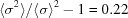. After covering by a silica shell, the particle diameter was determined by SAXS. Due to the different scattering-length densities of the cobalt ferrite and the silica, a simultaneous analysis of the size of the core and the shell was possible. The fit of the SAXS data resulted in a magnetic core diameter of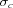= 146 ± 10 Å and an overall particle diameter of 712 ± 1 Å with an average polydispersity of p = 0.13. In the analysis a Schulz–Flory size distribution was assumed. Details about the core–shell data fit can be found elsewhere (Wagner, 2004).

Information on the particles' surface charges is contained in the effective number of charges used in the pair potential for two particles [equation (2)]. The interparticle correlations manifest themselves via a liquid-like structure factor S(Q), shown in Fig. 1. The structure factor of charge-stabilized particles can be fitted using the rescaled mean spherical approximation (RMSA; Hansen & Hayter, 1982). The solid line in Fig. 1describes the result of an RMSA fit to the experimental structure factor, yielding an effective charge Z = 250 e- and a volume fraction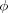= 0.027. The maximum of the structure factor is located at Q = 3.9 ×10-3 Å−1 implying a mean interparticle distance of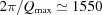Å.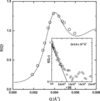Figure 1 Structure factor S(Q) (circles) and RMSA fit (line) of electrostatically interacting core–shell particles in suspension. The RMSA model gives an effective charge per particle of Z = 250   e- and a volume fraction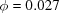. The insert displays the measured normalized intermediate scattering function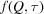at the Q value of the static structure-factor peak S(Qmax) where the solid line is a fit to the data in the short-time limit.

The size of the magnetic particles can also be verified by fitting the measured magnetization curves (Autenrieth, 2005). Fig. 2shows the normalized magnetization M/Msat (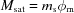, where ms is the saturation magnetization and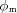is the volume fraction of the magnetic core) versus the applied magnetic field H of the core–shell particle suspension. The solid line displays the fit of the data using a Langevin model,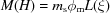with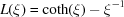and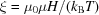, where kBT is the thermal energy. It results in a mean magnetic core diameter of= 143 ± 1 Å with a polydispersity of p = 0.24, in good agreement with the results of the scattering experiments.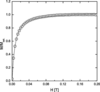Figure 2 Measured normalized magnetization of the magnetic particles with a silica shell versus the applied magnetic field H. The solid line is a fit to the data assuming the Langevin model.

Knowledge of the static behavior of the system is of prime importance, since the measured structure factor S(Q) is needed to evaluate the dynamics [equation (7)]. We note that the RMSA fit to the static data (Fig. 1) is excellent, thus indicating that the eventual effects of magnetic interactions do not (as expected) lead to significant influences on the static data as long as there is no external magnetic field involved.

The dynamic behavior was probed by means of XPCS over a largeQ range in order to investigate the Q dependence of the diffusion coefficient D(Q). Therefore, intensity correlation functions were measured at different Q values. Equation (5)was used to fit the short-time fall-off of the measured normalized intermediate scattering function in order to extract D(Q). An example is shown in the insert of Fig. 1, displaying the normalized intermediate scattering function and the corresponding short-time fit for the Q value of the peak of the structure factor S(Q).

The free diffusion coefficient D0 = 5.56 ×10-12 m2 s−1 is obtained from a highly diluted suspension by means of DLS. It does not show any Q dependence as the particles do not interact. In Fig. 3, the normalized inverse diffusion coefficient D0/D(Q) is compared to the static structure factor S(Q). The two observed peaks are located at the same value of Q indicating that the fluctuations of the most probable configuration decay the slowest. According to equation (7), the hydrodynamic function H(Q) can be extracted from the measured structure factor S(Q) and D0/D(Q). The result is shown in Fig. 4. The observed H(Q) is smaller than unity over the whole Q range. This indicates that the indirect interactions, mediated by the solvent, act as a friction force slowing down the dynamics of the system. The result obtained from purely experimental data is compared to a theoretical model. For charge-stabilized systems there is presently only one model available; the pairwise-additive approximation (PA) by Nägele & Baur. We used the pairwise-additive approximation to describe the hydrodynamic behavior of our suspension. The PA approach clearly overestimates the experimental data, as shown by the solid line in Fig. 4. We note, however, that the pairwise-additive approximation is a two-particle approach, thus limited to highly dilute systems. It is thus not surprising that the model fails to describe the data. There is no evidence that these deviations would be caused by magnetic effects since similar observations are reported also for non-magnetic particles (Riese, 2000; Grübel et al., 2000). There it is argued that the hydrodynamics of concentrated suspensions of highly-charged particles show resemblance to hard-sphere hydrodynamics, however with an additional retardation caused by the charge-stabilized character of the systems. Similar observations are also reported by Robert (2001). The origin of the observed retardation is presently unknown. At present we are led to conclude that there is no model available that can describe the hydrodynamic behavior of highly-charged colloidal suspensions at intermediate concentration.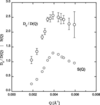Figure 3 Normalized inverse diffusion coefficient D0/D(Q) and static structure factor S(Q).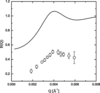Figure 4 Hydrodynamic function H(Q) for the core–shell particle suspension with volume fraction= 0.027. The solid line shows the prediction of the pairwise-additive approximation.

### 4. Conclusion

We synthesized a colloidal suspension consisting of magnetic charge-stabilized core–shell nanoparticles. This fluid acts as a model system to investigate the dynamic behavior, especially in terms of long-range electrostatic interactions of the particles in suspension.

The particles have been characterized by several scattering methods (SAXS, DLS) and magnetization measurements in order to obtain information on the size and size distribution, the surface charges and the magnetic properties. We show that the surface charges and the magnetic properties have only to be taken into account if one applies an external magnetic field.

The dynamic behavior of a concentrated opaque suspension has been probed by X-ray photon correlation spectroscopy. From the measured structure factor S(Q), obtained by SAXS, and the diffusion coefficients D0 and D(Q) the hydrodynamic function H(Q) could be extracted.

The attempt to describe the hydrodynamic function H(Q) within the framework of the pairwise-additive approximation failed and points to the need for an appropriate theoretical model. Supporting experimental studies are in progress.

### Acknowledgements

We thank Birgit Fischer for assistance during the experiments and Wolfram Härtl for fruitful discussions. The present work was supported by the Deutsche Forschungsgemeinschaft in the framework of the priority program SPP 1104.

### ReferencesJOURNAL OFAPPLIEDCRYSTALLOGRAPHY